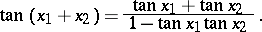# Tangent

(diff) ← Older revision | Latest revision (diff) | Newer revision → (diff)

The trigonometric function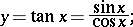another notation is: tg. Its domain of definition is the entire number axis with the exception of the points,. The tangent is an unbounded, odd and periodic (withas the smallest positive period) function. The tangent and the cotangent are connected by the relation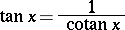The inverse function to the tangent is called the arctangent.

The derivative of the tangent is: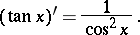The indefinite integral of the tangent is: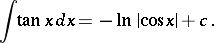The tangent has a series expansion: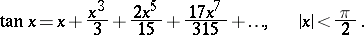The tangent of a complex argumentis a meromorphic function with zeros at the points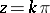, where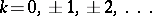.

The general term in the series expansion of the tangent is: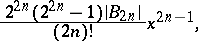whereare the Bernoulli numbers.